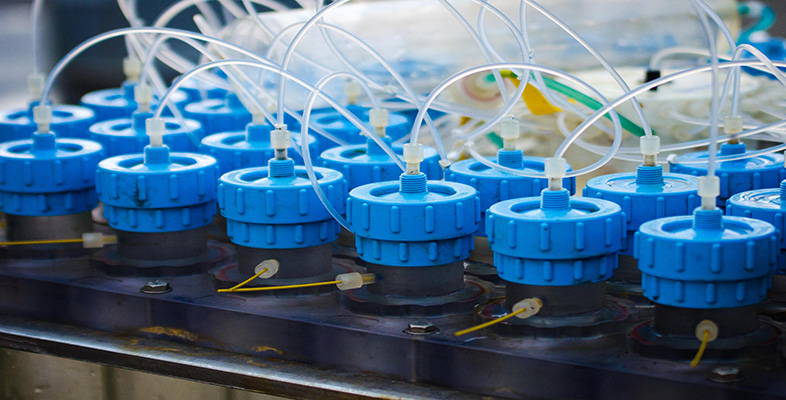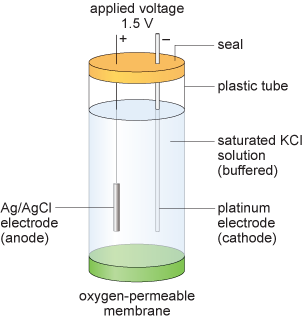Test kits for water analysis

Start this free course now. Just create an account and sign in. Enrol and complete the course for a free statement of participation or digital badge if available.

Free course

# 6.1.2 Amperometric DO probes

The DO probe described in Section 6.1.1 works rather like a battery in that a current is produced by the electrochemical reactions in solution.

An alternative approach is to use the amperometric principle, whereby a constant voltage is applied to an electrolysis cell, and the current flowing through the circuit is measured. An amperometric method of measuring DO was developed by Leland Clark in 1956. Current models have two electrodes immersed in a buffered saturated aqueous potassium chloride solution (Figure 9) housed within a plastic tube with an oxygen permeable membrane.Figure 9  The main components of an amperometric dissolved oxygen probe.

At the anode, which is typically a silver/silver chloride electrode, the following reaction occurs:

Cl (aq) + Ag(s) = AgCl(s) + e
Equation label: (19)

The reaction at the cathode, which is typically a platinum wire, is

O2 (g) + 4H+(aq) + 4e = H2O(l)
Equation label: (20)

A constant voltage of about 1.5 V is applied across the electrodes. When placed in a water sample, oxygen will diffuse through the membrane to the platinum electrode, and due to the applied voltage, will be reduced. The magnitude of the current in the circuit is directly proportional to the oxygen concentration of the sample.

S230_1